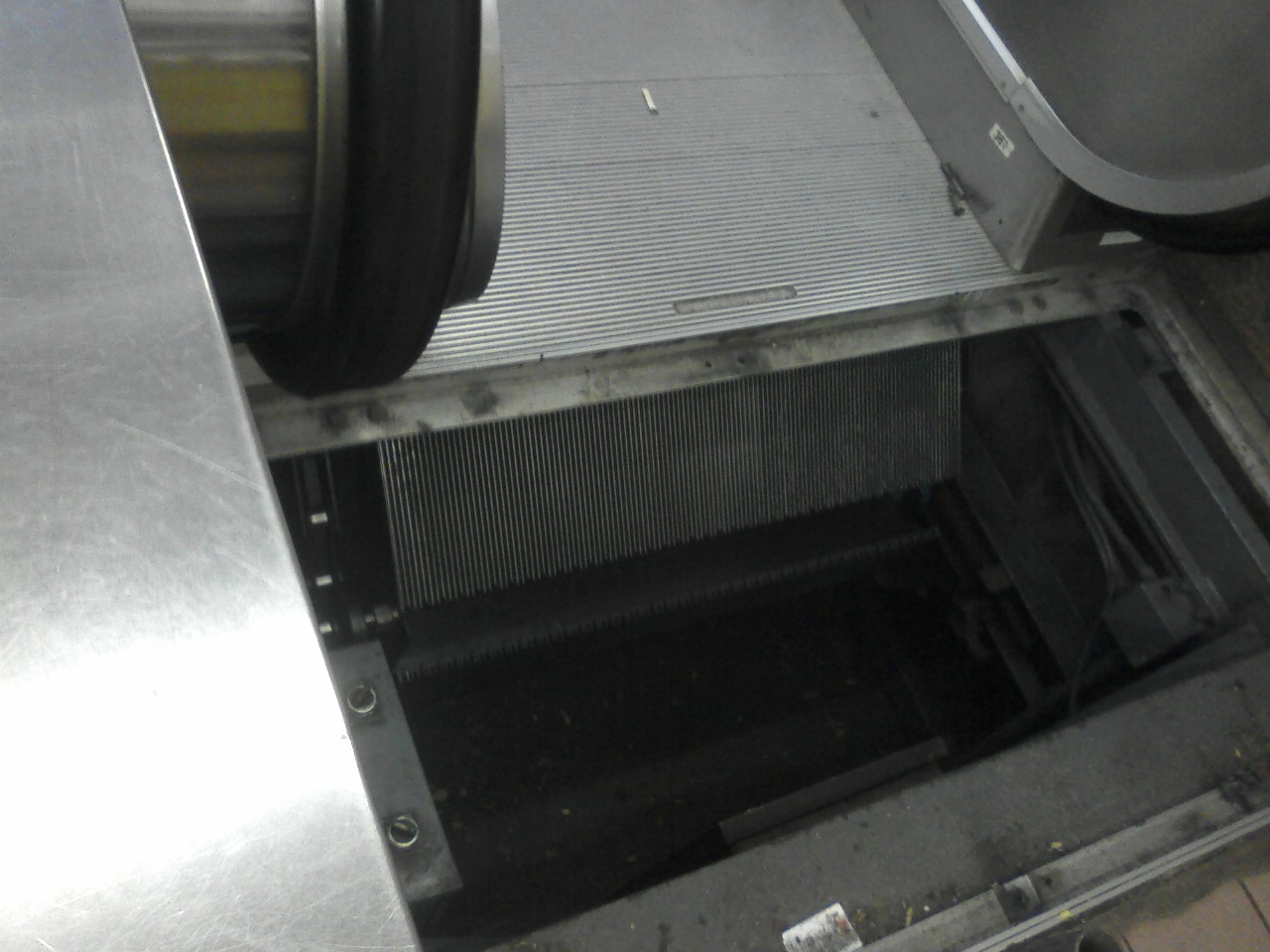### a polynomial problem

The latest problem from fakalin. Took some wrong turns and hints to solve it…

Given a polynomial $$f: \mathbb{Z}\to \mathbb{Z}$$ with positive integer coefficients, how many evaluations of $$f$$ does it take to obtain the polynomial?

(An $$f: \mathbb{R}\to \mathbb{R}$$ polynomial with real coefficients would take the number of degrees plus 1 to specify, which, if it held in this case, would render the answer unbounded. But the correct answer in this case is surprisingly small.)

### escalator

How do escalators work? I’ve wondered for years how escalators recycled their step blocks internally. At one point I thought they slid past each other on all four faces to save on turning radius (because I thought the blocks locked along grooves). Today I see an escalator under repair. Now the answer is clear. It’s much simpler than that: the blocks just turn along a track in the most obvious way imaginable.### a card problem

Here is a problem quoted from fakalin. A full deck of cards has 52 cards. Suppose 10 of them were face up and 42 were face down. You are in a dark room holding the deck. How do you rearrange the deck into two subdecks so that they have the same number of cards facing up?

### can you tell Asians apart?

There is a Chinese, a Japanese, and a Korean in here. Identify them.